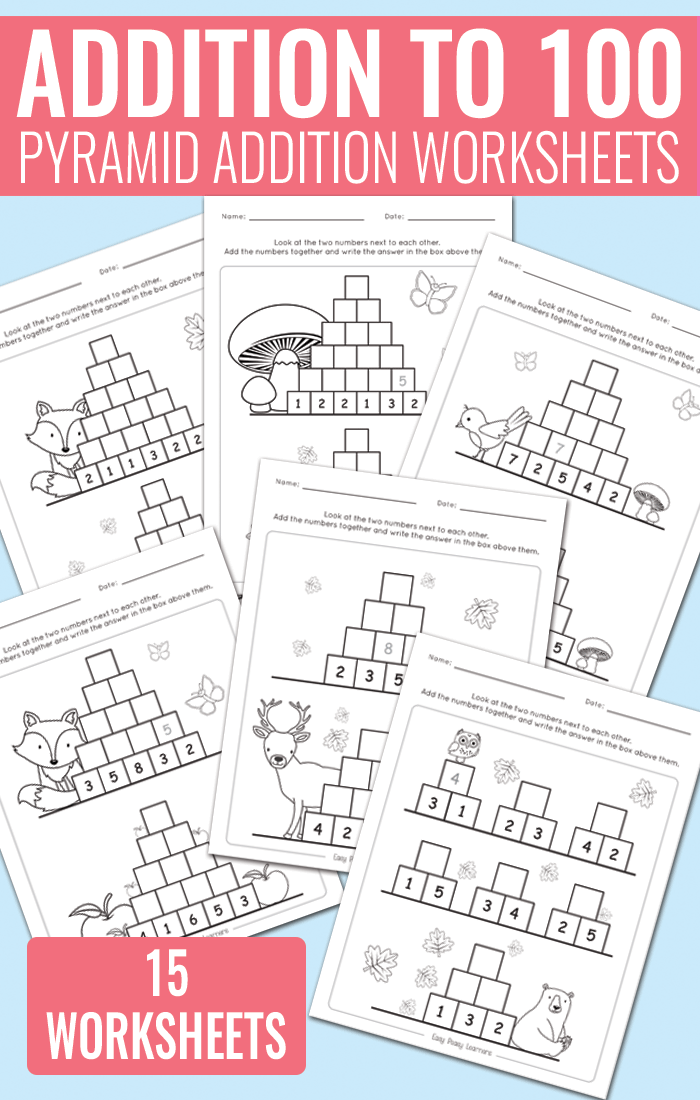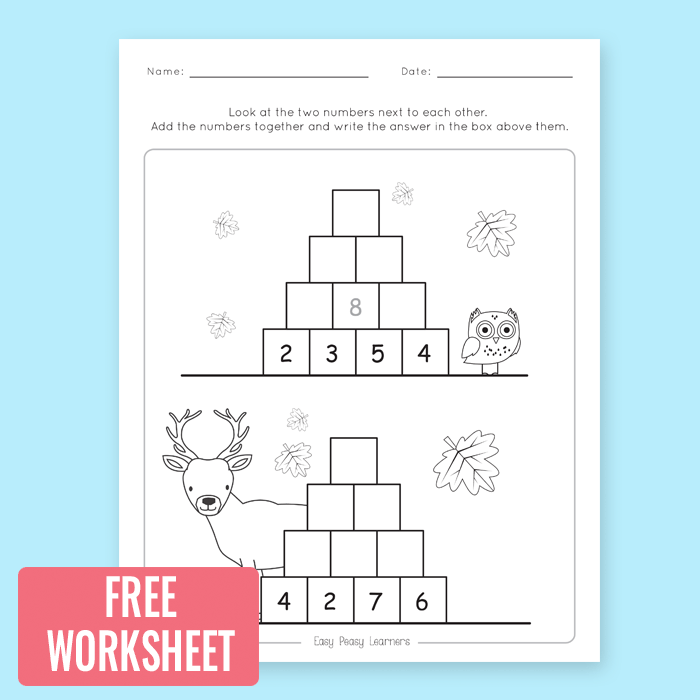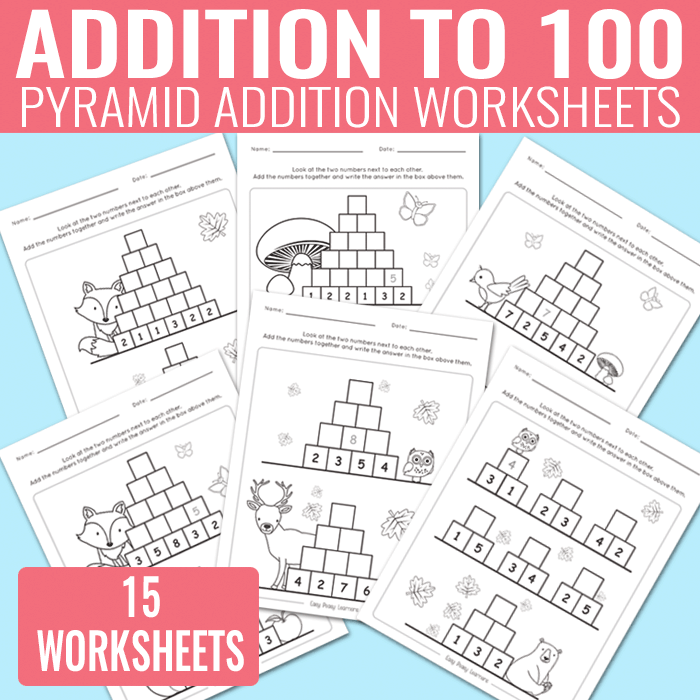# Pyramid Addition up to 100 Worksheets

Looking for a unique resource for addition practice? Give these pyramid addition up to 100 worksheets a try.## Pyramid Addition up to 100 Worksheets

There are 15 different worksheets in this printable learning pack

What you will get in this printable worksheet set;

• 15 unique addition up to 100 worksheets
• each worksheet has more than one pyramid to solve
• there are instructions on all pages and there is one equation solved on each of the pages

How are these solved? Students are asked to look at the two numbers in squares next to each other and add them up. They write down the answer into a square above those two squares. They continue solving the equations until they reach the top of the pyramid.

You can get the whole set at Teachers Pay Teachers where you can also preview the whole set.

Get Addition up to 100 Worksheets

Get Addition up to 100 Worksheets

These worksheets can be laminated for continuous use.Want to give these a try? You can grab one of the pages for free

Get Addition up to 100 Worksheets

More Basic Math Learning Resources

I Have, Who Has Addition and Subtraction Game

Skip Counting Worksheets (from 2 to 12)